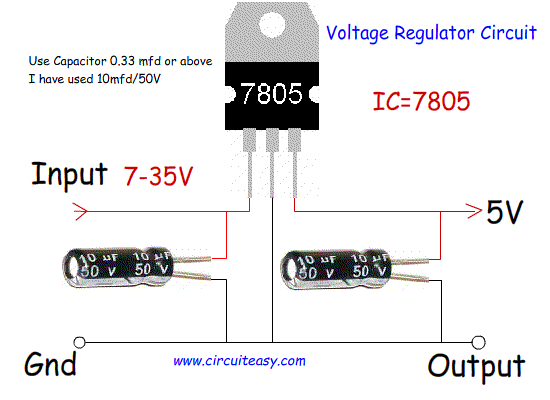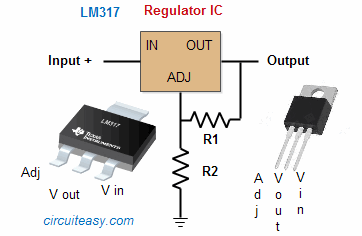top of page

# 12V to 5V 9V Voltage Converter using IC 7805, IC 7809

## DC Voltage Converter

In the field of electronics and experiment you will require to decrease voltage of a given supply for proper functioning of a device and for its safety. Here is a low cost solution using few components. We will use regulator IC such as 78xx.  This type of IC has 3 pin and is a positive voltage regulator. It has Internal Thermal Overload Protection, Short Circuit Protection. Last two digit of IC denotes its maximum output voltage.

for eg.

LM7805 = 5V output (widely used)

LM7806 = 6V output

LM7808 = 8V output

LM7809 = 9V output

LM7810 = 10V output

LM7812 = 12V output (widely used)

Note:Maximum current output is 1.5A, Minimum Input voltage should be 2V higher then the output Voltage.

12v to 5v, 9v, 12v DC converter circuitCircuit diagram of DC voltage regulator using 78XX.

Use of capacitor may be avoided in some circuit. You can use any capacitor 0.33 mfd or above. I have used 10mfd/50V on both side.

Working:

This type of regulator keeps on varying its resistance between input and output according to the Input voltage. These devices begins conducting and will conduct as much current as required to hold its output terminal voltage to that specified voltage by providing excess current.

Disadvantage of this type of regulator is energy is lost to maintain resistance in the form of heat. Input voltage should be greater than output atleast 2 Volt.

In the above circuit you saw that for every specific voltage you have to use different IC. Here is a solution for that is using programmable regulated power supply using IC such as IC LM317, LM117, LM338 etc.

Output is determined by the combination of two resistance.For Calculation of resistance and output voltage use :->  Calculator for Regulator LM317

* LM317 IC is widely used in Inverter, UPS etc.

bottom of page